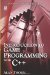# 19.3 SceneNode Properties and Methods

## 19.3 SceneNode Properties and Methods

Aside from sustaining a scene hierarchy and containing other nodes, SceneNodes can affect the objects they contain. SceneNodes can be moved, scaled, and rotated, which affects all attached child Entities. Likewise, SceneNodes can have their visibility status set to true or false, effectively hiding and unhiding all attached Entities. Following is a list of some of the methods of SceneNode that allow transformation.

• void scale (const Vector3 &scale)

Scales the node, combining its current scale with the passed-in scaling factor.

• void scale (Real x, Real y, Real z)

Scales the node, combining its current scale with the passed-in scaling factor.

• void translate (const Vector3 &d, TransformSpace relativeTo=TS_PARENT)

Moves the node along the Cartesian axes.

• void translate (Real x, Real y, Real z, TransformSpace relativeTo=TS_PARENT)

Moves the node along the Cartesian axes.

• void translate (const Matrix3 &axes, const Vector3 &move, TransformSpace relativeTo=TS_PARENT)

Moves the node along arbitrary axes.

• void translate (const Matrix3 &axes, Real x, Real y, Real z, TransformSpace relativeTo=TS_PARENT)

Moves the node along arbitrary axes.

• void roll (const Radian &angle, TransformSpace relativeTo =TS_LOCAL)

Rotates the node around the z-axis.

• void pitch (const Radian &angle, TransformSpace relativeTo=TS_LOCAL)

Rotates the node around the x-axis.

• Void rotate (const Vector3 &axis, const Radian &angle, TransformSpace relativeTo=TS_LOCAL)

Rotates the node around an arbitrary axis.

• Void rotate (const Quaternion &q, TransformSpace relativeTo=TS_LOCAL)

Rotates the node around an arbitrary axis using a quaternion.Introduction to Game Programming with C++ (Wordware Game Developers Library)
ISBN: 1598220322
EAN: 2147483647
Year: 2007
Pages: 225
Authors: Alan Thorn

Similar book on Amazon

flylib.com © 2008-2017.
If you may any questions please contact us: flylib@qtcs.net